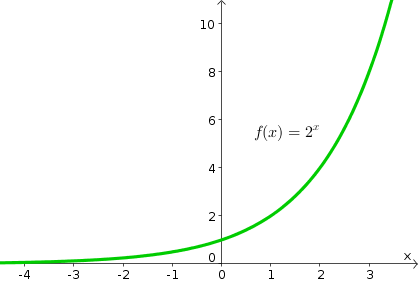# Math Insight

### Image: Exponential function $2^x$Graph of the exponential function $f(x)=2^x$.

Image file: exponential_function_two_to_x.png

Source image file: exponential_function_two_to_x.ggb
Source image type: Geogebra